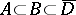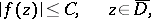# Ill-posed problems in complex function theory

(diff) ← Older revision | Latest revision (diff) | Newer revision → (diff)

Originally (Hadamard ill-posed) problems (cf. Ill-posed problems) for differential equations with a kind of instability similar to that of the Cauchy problem for the Laplace equation. For problems of this type one can construct the Hadamard example: to arbitrary small variations of the data together with such variations in any finite number of derivatives, correspond finite variations of the solutions (see , ). Nowadays the term "ill-posed problem" is understood in a considerably wider sense (see , ).

The problem of analytic continuation in the theory of functions of a (single) variable is ill-posed in the sense of Hadamard. For such functions the problem in its general form can be stated as follows. Given is a domainin the complex plane and two setsandbelonging to the closure of:. Onan analytic functionis given that is regular in. It is required to determineon. Concerning, apart from regularity in, there may be additional information, for example,(*)

whereis a given constant.

Classical problems concerning analytic continuation are: 1)is a subdomain of a domain; 2)is a part of the boundaryof; it is assumed thatis a (piecewise-) continuously differentiable closed curve,,; or 3)is a set having a limit point inside.

Uniqueness theorems for the problems mentioned were proved in the 19th century. (They can be found in text books on the theory of functions of a complex variable.) Problem 2) is equivalent to the Cauchy problem for the Laplace equation.

Estimates on the stability of analytic continuation problems of the type 1) and 2) are called three-constants theorems (estimates on the change in the solution of the problem in terms of a change in the data under conditions of type (*)). Problems concerning analytic continuation are linear, and the universal methods of regularization of linear ill-posed problems are applicable to them (see , ). For the problem 2) special methods of regularization have been worked out, based on the construction of Carleman functions (see ).

Problems concerning analytic continuation are connected with a broad range of applied problems. Frequently it follows from physical laws that various physical fields are analytic functions in certain variables. It is required to determine from the values of the field on a certain set (on which measurements are taken) the field on a larger set. Some of these applied problems are listed below.

1) The problem of determining the gravitational and magnetic field above the surface of the Earth from the values of the field on the surface. This problem is used in the exploration of deposits of useful minerals.

2) The problem of determining the potential of the flow of an ideal fluid or the filtration of a constant electric current inside a certain body from the value of the flow or potential on part of the surface (see ).

3) The problem of determining a function of compact support from the values of its Fourier transform on a finite segment.

From the point of view of applications, the problem of determining an analytic function from its values on finite sets is of considerable interest. The solution of this problem is not unique. However, if the set is close in a certain sense to a set of uniqueness (cf. Uniqueness properties of analytic functions), an approximate solution with a small error is possible.

For functions of several complex variables there are both well-posed and ill-posed problems of analytic continuation. For problems of analytic continuation from sets inside a domain of regularity the following general result is known: For the set to be a set of uniqueness, it is necessary and sufficient that it is not the union of finitely many analytic manifolds.

How to Cite This Entry:
Ill-posed problems in complex function theory. Encyclopedia of Mathematics. URL: http://encyclopediaofmath.org/index.php?title=Ill-posed_problems_in_complex_function_theory&oldid=14039
This article was adapted from an original article by M.M. Lavrent'ev (originator), which appeared in Encyclopedia of Mathematics - ISBN 1402006098. See original article